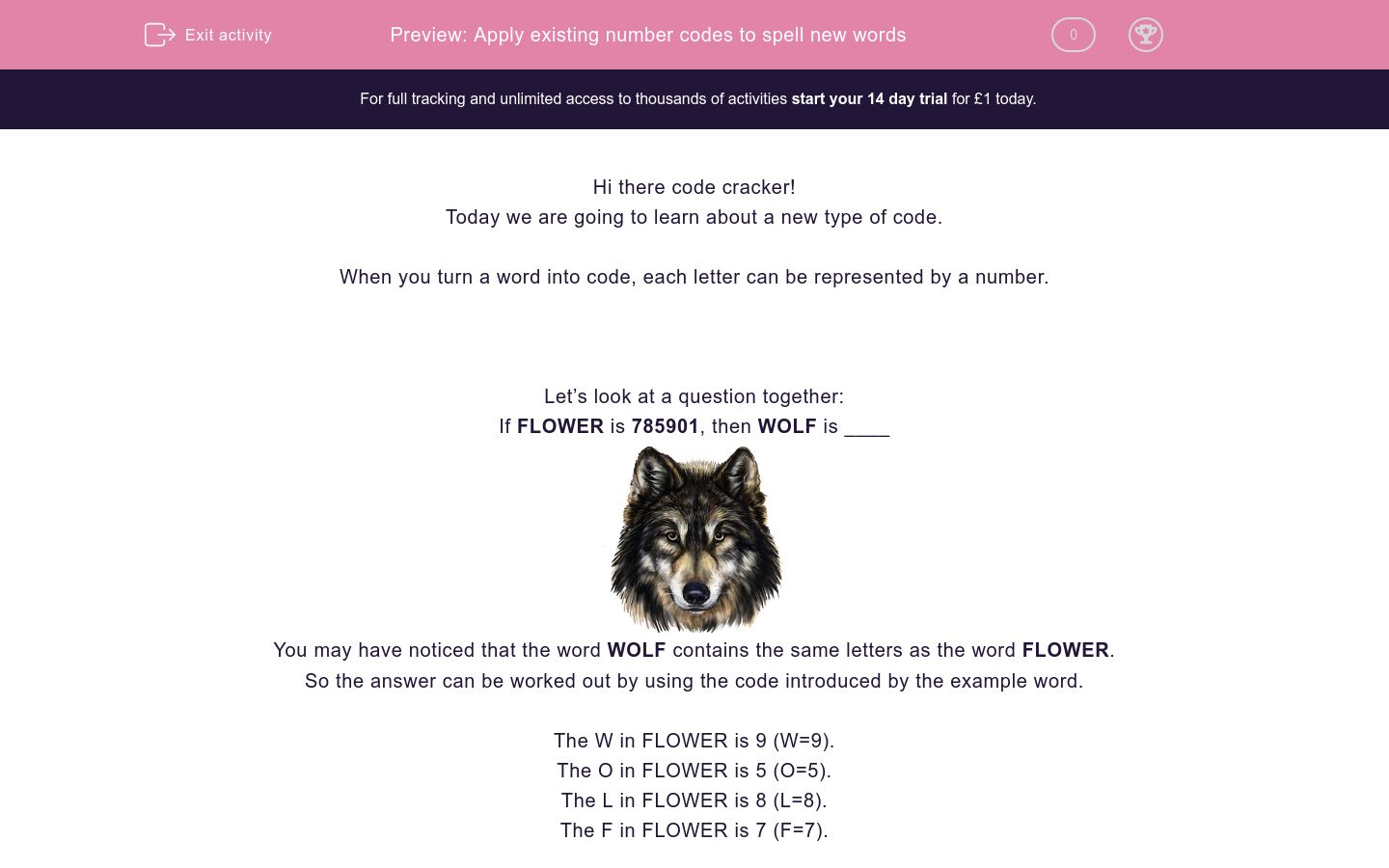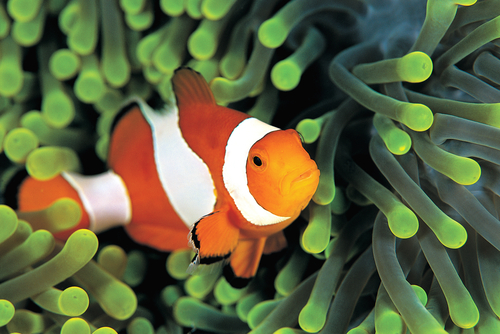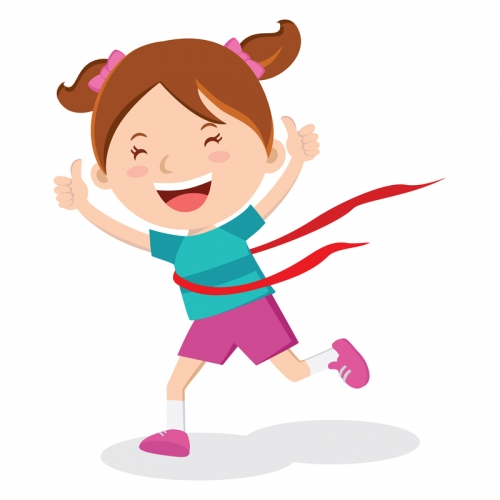# Apply existing number codes to spell new words

In this worksheet, students will turn words into number codes using words with similar codes as a base. It will develop their encoding and problem-solving skills.Key stage:  KS 2

Curriculum topic:  Verbal Reasoning

Curriculum subtopic:  Number Code Breaking

Difficulty level:### QUESTION 1 of 10

Hi there code cracker!

Today we are going to learn about a new type of code.

When you turn a word into code, each letter can be represented by a number.

Let’s look at a question together:

If FLOWER is 785901, then WOLF is ____You may have noticed that the word WOLF contains the same letters as the word FLOWER.

So the answer can be worked out by using the code introduced by the example word.

The W in FLOWER is 9 (W=9).

The O in FLOWER is 5 (O=5).

The L in FLOWER is 8 (L=8).

The F in FLOWER is 7 (F=7).

So WOLF is 9587 in code.

Let’s try another:

If the code for DINOSAUR is 51372894, then AROUND is ____

The word DINOSAUR contains the same letters as AROUND, so we can use this to work out the new code.

So the code for AROUND is 847935.

In this activity, you will be a code cracker and change words into number codes. Make a note of the number that matches each letter; you may want to create your own grid to help with this.

Pssstt!! Here’s a handy hint to help you reach superstar status:

It can be really helpful to write the word out and then write the corresponding numbers above each letter.  Give it a go!

Let’s get started!

Hello, code cracker! I hope you are ready for some Year 4 level number code cracking!If the code for FLOWER is 261378, what is the code for LOW?

613

783

261

Are you ready to have another go, code cracker? Here we go!

If we know that the code for POSTING is 9876452, then what is the code for TOPS?Have you spotted, Nemo, code cracker?!

Right then, if we know that the code for FINISH is 246413 can you work out the code for FISH?

1364

2641

2413

OK, code cracker, let's keep working at these code cracking skills! You're making great progress so far!Right, if you know that the code for WANTED is 823461, what is the code for DENT?

We are almost halfway code cracker - well done!

So, this time we are going to be thinking about food!Right, if the code for BOLOGNESE is 421267595, what is the code for BONE?

4275

5274

2745

We are over halfway, code cracker - brilliant stuff!

Do you remember fidget spinners?! They came to my mind when I was writing this question...!

If the code for FIDGET is 913425, what is the code for DIG?

We are definitely making progress, code cracker, well done you!

So, let's think about nature for this question!If the code for DAFFODIL is 43771498, what is the code for LID?

OK, code cracker, only a few questions to go now!If the code for NUMBER is 751234, what is the code for RUN?

754

457

547

You are almost at the end, code cracker. That's brilliant work!Right then, what do we think the code for HUG might be if the code for COUGH is 97184?

You have made it to the finish line, code cracker - what a champion!So, let's have a final go at cracking those codes!

If the code for LOVING is 967423, what is the code for NIL?

• Question 1

Hello, code cracker! I hope you are ready for some Year 4 level number code cracking!If the code for FLOWER is 261378, what is the code for LOW?

613
EDDIE SAYS
Did you get this one, code cracker? Don't worry if not - it is a bit of a tricker skill trying to work the codes out for yourself! The word LOW contains the same letters as the word FLOWER. We know that the code for the word FLOWER is 261378. So we can use this code to help us work out the code for LOW: The L in FLOWER is 6. The O in FLOWER is 1. The W in FLOWER is 3. So, if we put this together we see that the code for the word FLOWER is 613.
• Question 2

Are you ready to have another go, code cracker? Here we go!

If we know that the code for POSTING is 9876452, then what is the code for TOPS?

6897
EDDIE SAYS
You had to reorganise the letters in this one, code cracker. Did you spot that? We will keep using the same technique as before, though, so no need to panic! We know the code for the word POSTING and this contains the same letters as the word TOPS. If we use this code, we can work out that: The T in POSTING is 6. The O in POSTING is 8. The P in POSTING is 9. The S in POSTING is 7. So, the code for TOPS must be 6897. I hope that makes sense, code cracker; let's practise your skills on another question!
• Question 3Have you spotted, Nemo, code cracker?!

Right then, if we know that the code for FINISH is 246413 can you work out the code for FISH?

2413
EDDIE SAYS
Let's see how we got to this answer then, code cracker! Have you been using your handy hint to help you reach superstar status? Remember: write the word out and then write the corresponding numbers above each letter. It can really help! So, we know that the code for FINISH is 246413. From this, we can work out: The F in FINISH is 2. The I in FINISH is 4. The S in FINISH is 1. The H in FINISH is 3. If we put it all together, we get the code 2413, which must be the code for FISH!
• Question 4

OK, code cracker, let's keep working at these code cracking skills! You're making great progress so far!Right, if you know that the code for WANTED is 823461, what is the code for DENT?

1634
EDDIE SAYS
Are you getting the hang of this now, code cracker? I hope it's becoming a bit clearer and that you are using the handy hint to help you reach superstar status! Now, we know the code for WANTED is 823461 and that this word contains the same letters as the word DENT. So, let's break it down: The D in WANTED is 1. The E in WANTED is 6. The N in WANTED is 3. The T in WANTED is 4. So, the code for DENT must be 1634! Let's keep at it, code cracker!
• Question 5

We are almost halfway code cracker - well done!

So, this time we are going to be thinking about food!Right, if the code for BOLOGNESE is 421267595, what is the code for BONE?

4275
EDDIE SAYS
Did you get this one right, code cracker? BOLOGNESE is quite a long word, so don't worry if not. I'll show you how to get there now! I would definitely recommend writing out the word BOLOGNESE and writing the code above it, so that you know which number corresponds with which letter. If we do that, we can get to the code for BONE much more easily. We'll see that: The B in BOLOGNESE is 4. The O in BOLOGNESE is 2. The N in BOLOGNESE is 7. The E in BOLOGNESE is 5. So, we get the code 4275 for the word BONE.
• Question 6

We are over halfway, code cracker - brilliant stuff!

Do you remember fidget spinners?! They came to my mind when I was writing this question...!

If the code for FIDGET is 913425, what is the code for DIG?

314
EDDIE SAYS
Did you get to 314? Let's use our tried and tested technique to help us: writing the code above the word! If we do that, we can see that: The D in FIDGET is 3. The I in FIDGET is 1. The G in FIDGET is 4. So, the code for DIG is 314!
• Question 7

We are definitely making progress, code cracker, well done you!

So, let's think about nature for this question!If the code for DAFFODIL is 43771498, what is the code for LID?

894
EDDIE SAYS
Although the word DAFFODIL is a long one, remember that you only have to find the code for LID - this is nice and short and just the last three letters of the word DAFFODIL backwards! I really hope you wrote this one out to help you. If you didn't, try doing that now. So, we know that the code for DAFFODIL is 43771498. So, we know: The L in DAFFODIL is 8. The I in DAFFODIL is 9. The D in DAFFODIL is 4. So, the code for LID is 894. I hope that makes sense now!
• Question 8

OK, code cracker, only a few questions to go now!If the code for NUMBER is 751234, what is the code for RUN?

457
EDDIE SAYS
OK, did you manage this one? If you are finding that you are matching the wrong numbers to the wrong letters, then make sure you are practising writing the word out and putting the code above or beneath it, to make sure the numbers and letters match up correctly. So, we know that the code for NUMBER is 751234. So: The R in NUMBER is 4. The U in NUMBER is 5. The N in NUMBER is 7. Which means that the code for RUN is 457. I hope that makes sense, code cracker!
• Question 9

You are almost at the end, code cracker. That's brilliant work!Right then, what do we think the code for HUG might be if the code for COUGH is 97184?

418
EDDIE SAYS
Right then, I think you're getting the hang of this now, code cracker! Let's just check to make sure you understand how we get to this answer. If we know that the code for COUGH is 97184, then we know that: The H in COUGH is 4. The U in COUGH is 1. The G in COUGH is 8. So, the code for HUG must be 418!
• Question 10

You have made it to the finish line, code cracker - what a champion!So, let's have a final go at cracking those codes!

If the code for LOVING is 967423, what is the code for NIL?

249
EDDIE SAYS
Your last question, code cracker - wow! Well done, you! So, let's get to the bottom of this. Did you write out your words and numbers? It's always helpful to do so, remember that handy hint! We know that the code for LOVING is 967423, so from that we can work out that: The N in LOVING is 2. The I in LOVING is 4. The L in LOVING is 9. So, that gets you to the code 249 for NIL. You did it!
---- OR ----

Sign up for a £1 trial so you can track and measure your child's progress on this activity.

### What is EdPlace?

We're your National Curriculum aligned online education content provider helping each child succeed in English, maths and science from year 1 to GCSE. With an EdPlace account you’ll be able to track and measure progress, helping each child achieve their best. We build confidence and attainment by personalising each child’s learning at a level that suits them.

Get startedStart your £1 trial today.
Subscribe from £10/month.